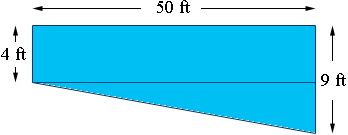SEARCH HOMEMath Central Quandaries & QueriesSubject: cubic feet Name: Steve Who are you: Parent how do we measure the cubic feet of a pool. The pool is 50 feet long by 40 feet wide by 4 feet at the shallow end and 9 feet at the deep end. Thank youHi Steve,

I drew a cross section of the pool assuming that there is a constant slope from 4 ft to 9 ft deep. The volume of the pool is the area of this cross section time the width of 40 ft.I drew a line on the diagram to divide it into a rectangle of length 50 ft and height 4 ft and a triangle with base 50 ft and height 9 - 4 = 5 ft. The area of the cross section is then the sum of the areas of the rectangle and the triangle.

PennyMath Central is supported by the University of Regina and The Pacific Institute for the Mathematical Sciences.